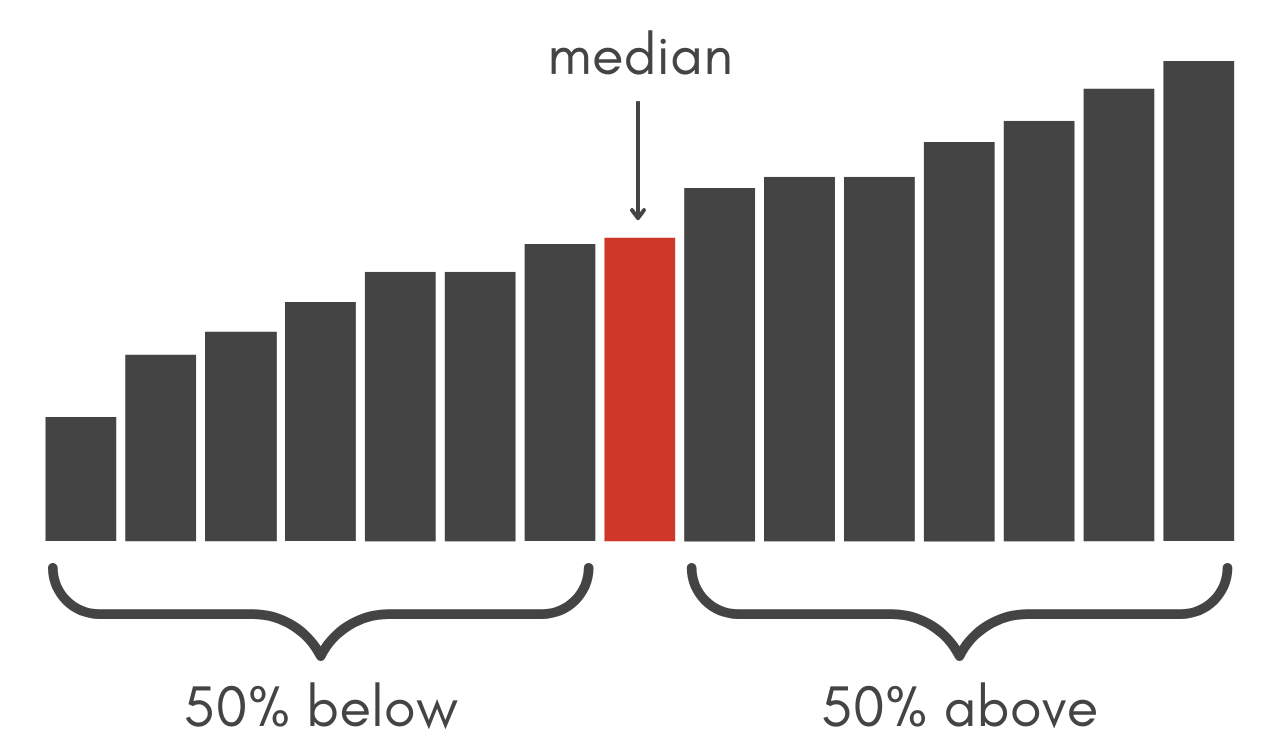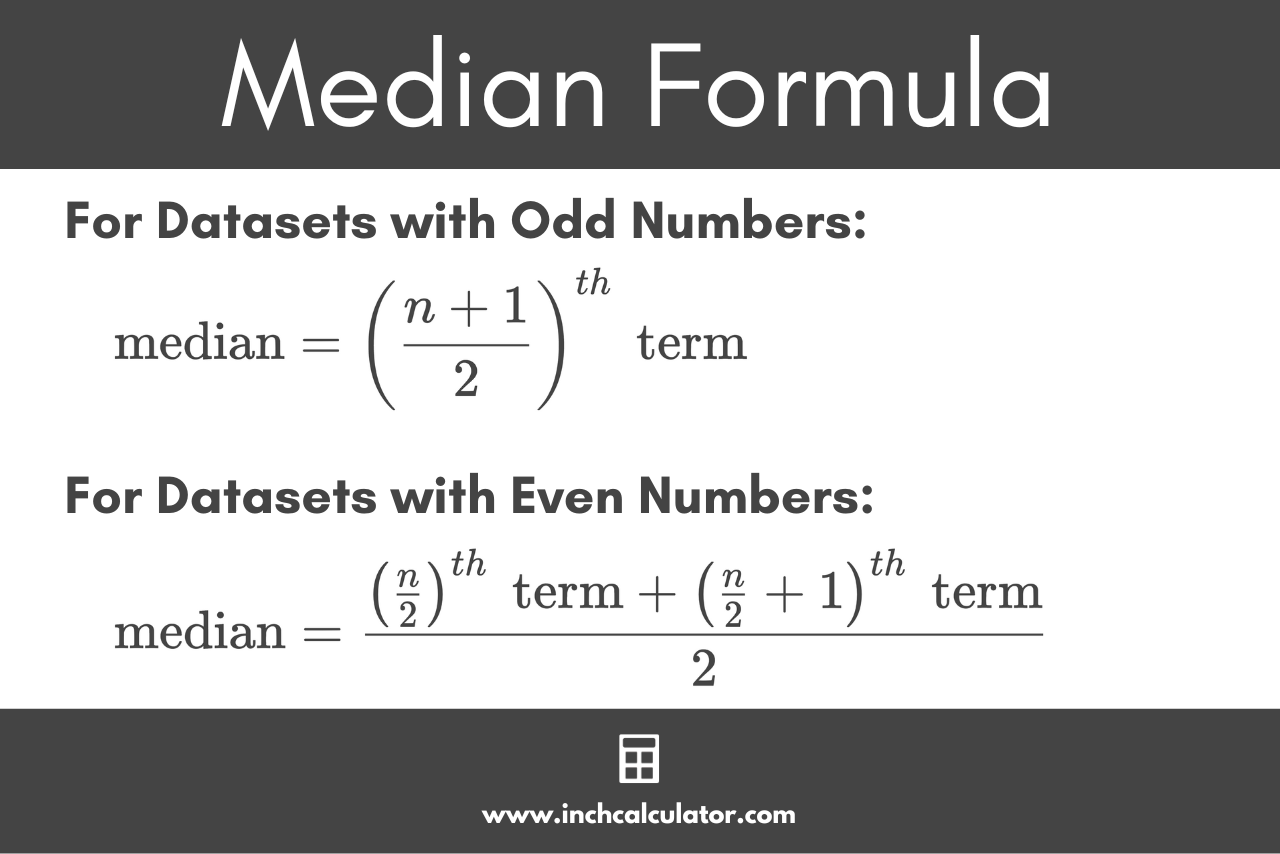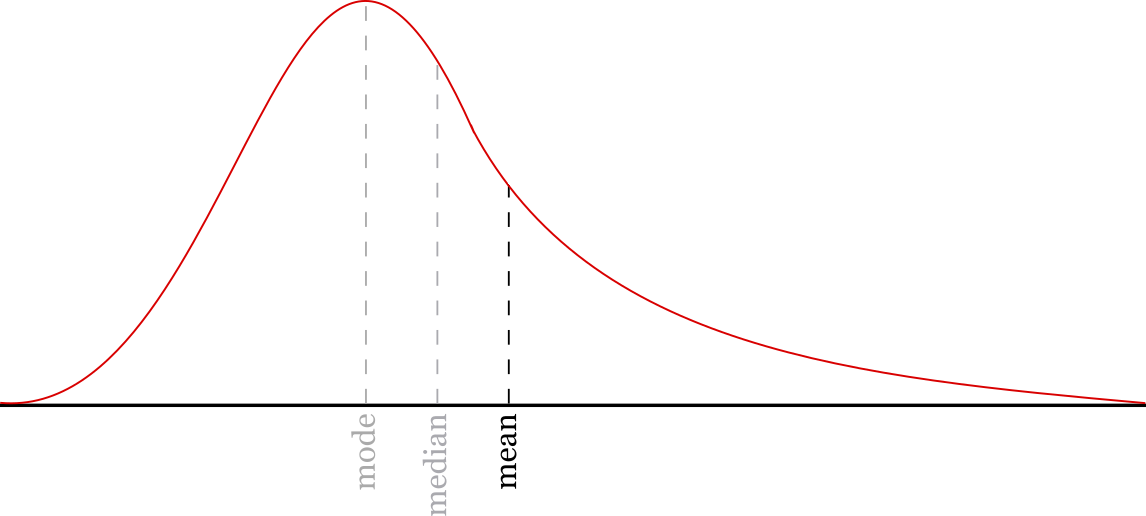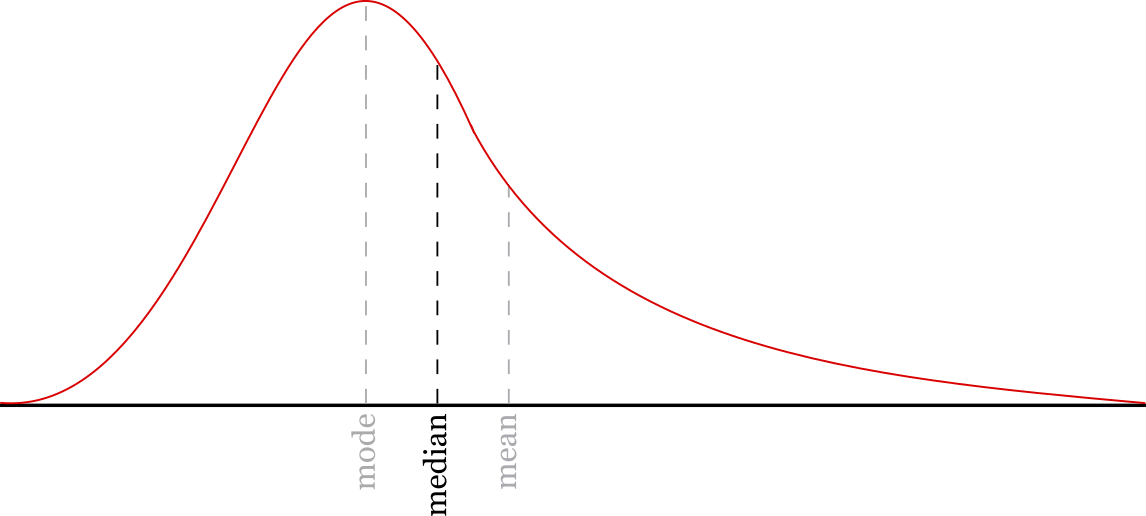# Median Calculator

Calculate the median of a number set using the calculator below.

Separate numbers using a comma (,)

## Results:

### Sorted Data:

Learn how we calculated this below

## How to Find the Median

The median is the middle value of a dataset and is a way to express the midpoint of a set of numbers. Or rather, it’s a measure of the central tendency of numeric data.

Essentially, the median is a way to express the middle, or center, of a set of numbers. Half of the numbers will be higher than the median and half will be lower.Before you can find the median of a data set, it needs to be sorted in ascending order. Sort the numbers from lowest to highest, then count the numbers in the set.

### Median Formula

Since the process of finding the median is different for datasets with odd and even numbers, there are two formulas to find the median.

#### Formula for Median for Datasets With Odd Numbers

You can find the median for a sorted dataset with an odd number of values using the following formula:

\text{median}=\left ( \frac{n + 1}{2} \right )^{th}\text{ term}

The median is equal to the (n + 1)/2th term in the dataset, which is equal to the number at the index of the count of the data n plus 1, divided by 2.

If the count of the numbers is odd, then the median is the number exactly in the middle of the data.

Find the middle index of the numbers by adding one to the count and then dividing by 2. The result is the index of the median in the dataset.

#### Formula for Median for Datasets With Even Numbers

You can find the median for a sorted dataset with an even number of values using the following formula:

\text{median}=\frac{\left ( \frac{n}{2} \right )^{th}\text{ term} + \left ( \frac{n}{2} + 1 \right )^{th}\text{ term}}{2}

The median is equal to the n/2th term plus the (n/2) + 1th term, divided by 2.

If the count of the numbers is even, then the median is actually the average of the middle two numbers in the data. Find the two numbers in the middle by dividing the count by 2 to find the lower-middle index, then adding 1 to find the upper-middle index.

Then, add the numbers at the lower and upper-middle indices and divide the result by 2. The median for an even dataset is actually the mean of the middle two values.### Example: Finding the Median

Calculate the median of the following numbers: [3,7,2,9,5]

Step 1: sort the numbers.
[2,3,5,7,9]

Step 2: count the numbers
count = 5

Step 3: find the middle index
median = x(5 + 1)/2
median = x6/2
median = x3

Step 4: find the number at the index 3
[2,3,5,7,9]
median = 5

The median of the numbers above is 5.

## Median vs. Mean

The median is one of the observations of data used in summary statistics, but another commonly used metric to describe numeric data is the mean.

The mean is another measurement of the central tendency of a set of data. The mean is the average value of the data rather than the midpoint of the data.The mean represents the average value of a number set. It is equal to the sum of the data divided by the count of the numbers in the data.

So should you use the median or the mean to describe a set of data?

The median is a good way to find the middle of the data and ignore outlying data points that might skew the average result. It reflects the middle of the typical values of the data.The mean might be more useful if the average value of the entire set of data is important.

The mean considers each value in the data, so it might be a more accurate way to describe the central tendency of the data, especially if the majority of the data are in an evenly distributed range.

But, it’s important to note that major outliers in the data can skew the mean. Consider the data: [3,4,5,6,91]

The average of this data is 21.8, and the median value is 5.

The median might do a better job of discarding those outliers and reducing the skew since it only considers the midpoint of the data. But, there are some cases when outlier values should be considered, making the mean a better representation of the data.

Our mean, median, & mode calculator offers even more statistical observations for your data.